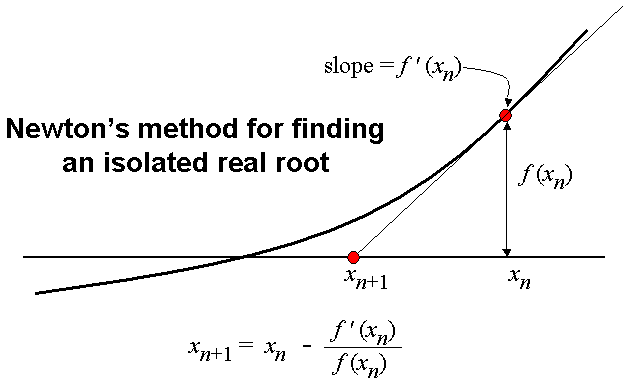## NEWTON RAPHSON METHOD:Program to Implement NEWTON RAPHSON METHOD to solve eqn.

## Coding:

#include<stdio.h>
#include<conio.h>
#include<math.h>
#include<string.h>
#include<process.h>

// Formulae Declaration
#define f(x)   3*x - cos(x) - 1
#define df(x)  3+sin(x)
int n;

void NEW_RAP();

void main()
{
clrscr();
printf("\n Solution by NEWTON RAPHSON METHOD ");
printf("\n\n Equation is -> 3*x - cos(x) - 1 = 0\n");
printf("\n Enter the no. of iterations ");
scanf("%d",&n);
NEW_RAP();
getch();
}

void NEW_RAP()
{
float x1,x0;
float f1,f0;
float df0;
int i=1,itr;
float EPS,error;

/* finding an approximate Root of a given equation, having +ve value*/
for(x1=0.0;;x1+=0.01)
{
f1=f(x1);
if(f1>0)
break;
}
itr=n;
x0=x1-0.01;
f0=f(x0);
printf("\n Enter the Maximum possible error: ");
scanf("%f",&EPS);
if(fabs(f0)>f1)
printf("\n\t\t The root is near to %.4f\n\n\n",x1);
if(f1>fabs(x0))
printf("\n\t\t The root is near to %.4f\n\n\n",x0);
x0=(x0+x1)/2;
for(;i<=itr;i++)
{
f0=f(x0);
df0=df(x0);
x1=x0-(f0/df0);
printf("\n\t\t The %d approximation to the root is : %f",i,x1);
error=fabs(x1-x0);
if(error<EPS)
break;
x0=x1;
}
if(error>EPS)
{
printf("\n\n\t NOTE :-");
printf(" The No. of Iterartions are not sufficient. ");
}
printf("\n\n\n\t\t----------------------------------------------------");
printf("\n\t\t ROOT  = %.4f ",x1);
printf("\n\t\t----------------------------------------------------");
}
NEWTON RAPHSON METHOD in CReviewed by Tanuj on 7:30:00 am Rating: 5